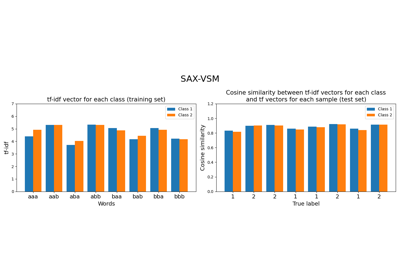# `pyts.classification`.SAXVSM¶

class `pyts.classification.``SAXVSM`(window_size=0.5, word_size=0.5, n_bins=4, strategy='normal', numerosity_reduction=True, window_step=1, threshold_std=0.01, norm_mean=True, norm_std=True, use_idf=True, smooth_idf=False, sublinear_tf=True, overlapping=True, alphabet=None)[source]

Classifier based on SAX-VSM representation and tf-idf statistics.

Time series are first transformed into bag of words using Symbolic Aggregate approXimation (SAX) algorithm followed by a bag-of-words model. Then the classes are transformed into a Vector Space Model (VSM) using term frequencies (tf) and inverse document frequencies (idf).

Parameters: window_size : int or float (default = 0.5) Length of the sliding window. If float, it represents a percentage of the size of each time series and must be between 0 and 1. word_size : int or float (default = 0.5) Length of the words. If float, it represents a percentage of the length of the sliding window and must be between 0. and 1. n_bins : int (default = 4) The number of bins to produce. It must be between 2 and `min(window_size, 26)`. strategy : ‘uniform’, ‘quantile’ or ‘normal’ (default = ‘normal’) Strategy used to define the widths of the bins: ‘uniform’: All bins in each sample have identical widths ‘quantile’: All bins in each sample have the same number of points ‘normal’: Bin edges are quantiles from a standard normal distribution numerosity_reduction : bool (default = True) If True, delete sample-wise all but one occurence of back to back identical occurences of the same words. window_step : int or float (default = 1) Step of the sliding window. If float, it represents the percentage of the size of each time series and must be between 0 and 1. The step of sliding window will be computed as `ceil(window_step * n_timestamps)`. threshold_std: float (default = 0.01) Threshold used to determine whether a subsequence is standardized. Subsequences whose standard deviations are lower than this threshold are not standardized. norm_mean : bool (default = True) If True, center each subseries before scaling. norm_std : bool (default = True) If True, scale each subseries to unit variance. use_idf : bool (default = True) Enable inverse-document-frequency reweighting. smooth_idf : bool (default = False) Smooth idf weights by adding one to document frequencies, as if an extra document was seen containing every term in the collection exactly once. Prevents zero divisions. sublinear_tf : bool (default = True) Apply sublinear tf scaling, i.e. replace tf with 1 + log(tf). overlapping : bool (default = True) If True, time points may belong to two bins when decreasing the size of the subsequence with the Piecewise Aggregate Approximation algorithm. If False, each time point belong to one single bin, but the size of the bins may vary. alphabet : None or array-like, shape = (n_bins,) Alphabet to use. If None, the first n_bins letters of the Latin alphabet are used.

References

  P. Senin, and S. Malinchik, “SAX-VSM: Interpretable Time Series Classification Using SAX and Vector Space Model”. International Conference on Data Mining, 13, 1175-1180 (2013).

Examples

```>>> from pyts.classification import SAXVSM
>>> X_train, X_test, y_train, y_test = load_gunpoint(return_X_y=True)
>>> clf = SAXVSM(window_size=64, word_size=12, n_bins=5, strategy='normal')
>>> clf.fit(X_train, y_train)
SAXVSM(...)
>>> clf.score(X_test, y_test)
1.0
```
Attributes: classes_ : array, shape = (n_classes,) An array of class labels known to the classifier. idf_ : array, shape = (n_features,) , or None The learned idf vector (global term weights) when `use_idf=True`, None otherwise. tfidf_ : array, shape = (n_classes, n_words) Term-document matrix. vocabulary_ : dict A mapping of feature indices to terms.

Methods

 `__init__`([window_size, word_size, n_bins, …]) Initialize self. `decision_function`(X) Evaluate the cosine similarity between document-term matrix and X. `fit`(X, y) Fit the model according to the given training data. `get_params`([deep]) Get parameters for this estimator. `predict`(X) Predict the class labels for the provided data. `score`(X, y[, sample_weight]) Return the mean accuracy on the given test data and labels. `set_params`(**params) Set the parameters of this estimator.
`__init__`(window_size=0.5, word_size=0.5, n_bins=4, strategy='normal', numerosity_reduction=True, window_step=1, threshold_std=0.01, norm_mean=True, norm_std=True, use_idf=True, smooth_idf=False, sublinear_tf=True, overlapping=True, alphabet=None)[source]

Initialize self. See help(type(self)) for accurate signature.

`decision_function`(X)[source]

Evaluate the cosine similarity between document-term matrix and X.

Parameters: X : array-like, shape (n_samples, n_timestamps) Test samples. X : array-like, shape (n_samples, n_classes) osine similarity between the document-term matrix and X.
`fit`(X, y)[source]

Fit the model according to the given training data.

Parameters: X : array-like, shape = (n_samples, n_timestamps) Training vector. y : array-like, shape = (n_samples,) Class labels for each data sample. self : object
`get_params`(deep=True)

Get parameters for this estimator.

Parameters: deep : bool, default=True If True, will return the parameters for this estimator and contained subobjects that are estimators. params : dict Parameter names mapped to their values.
`predict`(X)[source]

Predict the class labels for the provided data.

Parameters: X : array-like, shape = (n_samples, n_timestamps) Test samples. y_pred : array-like, shape = (n_samples,) Class labels for each data sample.
`score`(X, y, sample_weight=None)

Return the mean accuracy on the given test data and labels.

Parameters: X : array-like, shape = (n_samples, n_timestamps) Univariate time series. y : array-like, shape = (n_samples,) True labels for X. sample_weight : None or array-like, shape = (n_samples,) (default = None) Sample weights. score : float Mean accuracy of `self.predict(X)` with regards to y.
`set_params`(**params)

Set the parameters of this estimator.

The method works on simple estimators as well as on nested objects (such as `Pipeline`). The latter have parameters of the form `<component>__<parameter>` so that it’s possible to update each component of a nested object.

Parameters: **params : dict Estimator parameters. self : estimator instance Estimator instance.

## Examples using `pyts.classification.SAXVSM`¶Symbolic Aggregate approXimation in Vector Space Model (SAX-VSM)